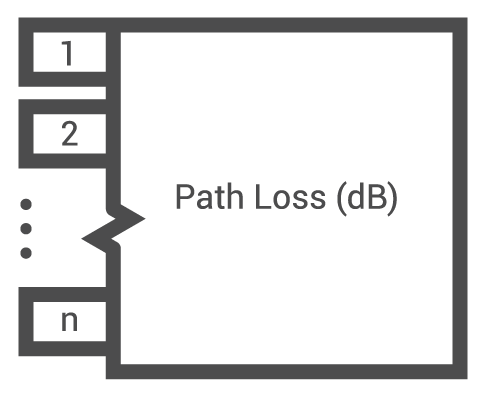# N-Way Power Divider Calculator

## This calculator determines the total path loss provided by an RF n-way power splitter.

(dB)

(dB)

### Overview

An N-way power divider, also known as a Wilkinson power divider, has become very popular in the RF engineering world because it allows isolation between the output ports while maintaining a matched condition on all ports. This tool will compute for the total loss in an N-way power divider given the total number of ports and the path loss. You can compute for up to 16 paths. Note that the path loss and total loss are presented in decibels (dB).### Equations

$$Loss_{total} = -10log_{10}(\frac{1}{N}) + Loss_{path}$$

Where:

$$N$$ = number of ports

### Applications

The Wilkinson power divider has many uses, particularly in RF technology. When you feed power to one port of a power divider, the power divides equally between all ports. The bidirectional nature of power dividers means you can also use them as power combiners.

Wilkinson power dividers are mostly seen in I/Q modulators & demodulators, high power transmission systems, and signal mixers. For example, a 5-way power divider can be used in multiple-channel superheterodyne receivers, to distribute the local oscillator signal to all channels at one time from a single source. The Wilkinson power divider can also be used in high power amplifiers and T/R modules for power combining.